# Pairing Lane Detection with Object Detection

As part of the Become a Self Driving Engineer nanodegree I’ve been doing for the past little bit, I was recently tasked with creating a Lane Detection Algorithm completely from scratch. It looks like this:

I wanted to take it up to a whole new level, so I slapped on some Object Detection to detect the cars in the gif above :)

# Motivation:

Object detection plays an integral role in the field of autonomous vehicle safety. The attempt I have made in this project is to develop a pipeline that can not only detect lane lines but also detect cars.

I do know the fact that state-of-the-art Deep Learning algorithms such as YOLO do exist but the motivation for this challenge was to develop a pipeline by implementing algorithms and using a non-typical Deep Learning approach. This allowed me to not only develop the math intuition behind these algorithms but also allow me to hardcode such algorithms instead of feeding the data straight into the neural network.

# Lane Detection Pipeline

The overall structure of the pipeline is as follows:

## Calibrate the camera using a chess/checkerboard to prevent distortion.

Cameras are not perfect. In the real world, using a camera that is distorted or warps the ends of its input can be fatal. Not being able to detect lane lines correctly and/or not detecting other cars is extremely dangerous. We can simply calibrate the camera by taking in the input image of a raw chessboard and calibrating it so that it undistorts.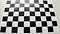Input, raw image of the camera taking a picture of a chessboard.

Notice that near the ends of the picture, the chessboard seems to get warped/curved.

What we can do to solve this is use OpenCV's cv2.findChessboardCorners function and then call cv2.calibrateCamera.

Once we run a script like this, our output of the undistorted camera would look like this: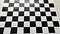Here’s how the camera looks after we undistort it.

Let’s take a look at how this would look from a self-driving car. Here’s our input image.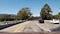Input image

Here’s how the camera image from the car looks after we undistort it. Pay close attention to the white car on the right side and the car on the opposite lane.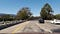Output image, after undistortion

Now that we’ve undistorted our image, we would now need to create a binary thresholded image using black and white pixels. This will allow for faster computation and will substantially reduce noise, allowing for the pipeline to track lane lines accurately.

## Creating a binary image using advanced colour transformation techniques

Now that we’ve undistorted our input camera feed, we’d now need to transform our image into a binary threshold scale (black and white, 0 = black and 1 = white).

This can be done using the Sobel filter. Otherwise known as the Sobel-Feldman operator, is an algorithm developed for computer vision; specifically in the use of computer vision and edge detection.

The filter is calculated by using a 3x3 kernel. This is then convolved to the original image to calculate the derivatives of the image itself. We’d have 2 separate kernel filters which are multiplied by the derivative of the x points with another one for the y points.

After that, we would then compute the gradient of the derivatives (which is a vector of its partial derivatives) for both x and y. After that, we’d take the magnitude of the gradients (take the square root of x²+y²).

What I did was that I thresholded the binary meaning that if the pixel value was in range (12,255) for x and (25,255) for y.

Now that we’ve coded it out, let’s take a look at our input image and its respective output. Here’s the input image:

And here’s the output image:

## Perspective transformation — getting that bird's eye view

What we’d do now in this step is warp our perspective. Instead of getting that front view from the camera placed at the front of the car, we can warp our perspective to a birds-eye view. This allows us to get rid of all the noise (cars, trees, etc.) and only focus on the actual lane lines themselves.

What we’d do is we would create a region of interest where we can assume that the lines are going to be. Because we already know that we won’t have lane lines at the top of the image or on either the left or right side, we can safely create our region of interest near the center in a trapezoidal manner (closer objects are bigger so we’d need to use a trapezoid and warp that.

Input image: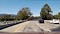Input image

Region of interest (our trapezoid):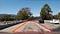Trapezoidal image

What we can do after is take in this region of interest, invert it, and then get our birds-eye view. cv2 has its own function, getPerspectiveTransform and warpPerspective. Using these 2 functions, we’re able to warp our perspective to the point that we get the birds-eye view.

Output image (after doing our perspective warp):

This is how our code would look like:

## The fun part — Lane Line Detection

Now that we’re really preprocessed our image and got the bird’s eye view, it’s time to detect lane lines!

What we can do to estimate and detect lane lines is use green boxes to estimate the polynomial. I used window sizes of 25x80px and fitted the lane lines against these. Here’s how it turned out:

Input image:

Output image:

But this isn’t accurate enough. We want a perfect line and curved distribution. What we can do is split the image in half (vertically) and make an array of all the points on the left and right sides where we have white pixels. Now that we’ve gotten the coordinate points for all the white pixels on the left and right side, we can use cv2.polyfit to fit the quadratic line of best fit and get the equation of the line. cv2.polyfit only returns the coefficients of Ax²+Bx+C (it only gives the A, B, and C), so we can simply just create the equation by taking the values and plotting them out.

Input image:Input image

Output image: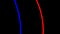We’ve gotten some really smooth, beautiful lines!!

## Boom! Lane Detection

Now, we can simply grab a video and fit our lane detection algorithm onto it!

# Part 2: Object Detection

Now that we’ve developed our lane detection algorithm, we’d now need to develop a pipeline for detecting cars and objects.

Here’s what our pipeline for this would look like:

## Data preprocessing

The first step in our object detection case would be to preprocess our data and get it ready to feed it in into our AI model. This would be done by taking a histogram of all the values in our image. In the real world, cars are not always the same size and vary in size by a fair amount. In template matching, the model is dependant on the raw colour values and the order of those values. That can vary substantially.

When we compute the histogram of colour values in an image, we’re able to compute the transformations that are robust to changes in appearance. When we do something like this, we are no longer dependant on the arrangement of every single pixel (whether it’s a car or not). The problem that occurs now is the fact that we’re now dependant on the colour of the car. For example, if I had a red car, I might have some really high values in the red channel of the image on which the model would train. If I, instead, had a blue car, the red channel values would be extremely low therefore classifying the car as “not a car.”

To solve this, we can compute the histogram of gradients. What this means is that we’d take our input image, compute the gradient of it. After that, we’d define a window size which would scan over the image (8x8 window size for example) and then group all the pixels within the 8x8 bucket (64 pixels) by taking the value of the gradient which has the highest magnitude.

The benefit of using such an extraction feature is the fact that our AI model would be robust and immune to variations in shape.

This is what a HOG of our Vehicle and Non-vehicle classes might look like:

Here’s a code snippet as to how you’d code that out:

## Building an AI model that can detect cars

Now that we’ve preprocessed our data, it’s ready to be fed into a model. I chose to develop a Support Vector Machine algorithm (which is a Supervised learning algorithm) to be able to detect vehicles and ones that are not. Note: remember that we are working with binary classes — one class for cars, the other class being a non-car (not a car).

Support Vector Machines make use of a hyperplane — a decision boundary to separate and distinguish between separate classes. In the case of support vector machines, a data/coordinate point would be considered as an n-dimensional vector. Our goal here is to figure out whether we can separate such points with an n-1 — dimensional hyperplane. The way that the hyperplane is decided is when we can have the maximum margin between classes, therefore reducing bias towards any side (this is also known as the maximum-margin hyperplane and the linear classifier is known as a maximum-margin classifier).

This is how our model training code would look like:

After training our model, we get a perfect accuracy of 100% (note that the model is not overfitting mainly because I also utilized a test set and reached accuracies > 93% on it).

## Creating a sliding window for object detection

Now that we’ve successfully trained our SVM model to detect vehicles vs non-vehicles, a sliding window algorithm would now have to be built in order to put the model into use.

Let’s say that we had a 64x64px window that we were scanning across the image. Whatever pixel values fall into that window, we’d then feed right into the SVM model and get its predictions. If the SVM model makes its prediction, we would draw a blue rectangle to indicate that.

This can be done using a HOG Sub-Sampling Window Search. We would specify the y-value ranges we would like our sliding window to be implemented in and specifically focus on that region for our object detection pipeline. This will allow faster computation time (which plays a significant role in the field of autonomous vehicles) and better predictions (since we’re not taking into account all the noise outside the image such as trees and the sky). Here’s how an input image might look like: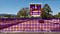Credit: Udacity

And now this region would be exactly where we’d want our predictions to be made.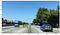Examples of car detection given this input image

Our code up until this point:

## False positives

What you might’ve noticed in the image above was the fact that we did have quite a few false positives and overlapping predictions. Since an algorithm such as this where there are multiple overlapping boxes is impractical, we’d want to eliminate false positives. This can be done by applying a heatmap and taking the average pixel values out of all the bounding boxes in order to get a stable prediction.

Wherever we get a positive detection, we will add a value of 1 to the pixel value, therefore, generating a heatmap. That would create an image like this:

Now what we’d do is threshold the images (if the pixel value is ≤ 0, then just make it 0). After that’d we’d simply draw the labelled boxes using this heatmap and boom! We’ve now gotten a reasonable car estimation.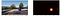Obviously, there is room for improvement but not bad!

# The final part: Putting this all together

Now that we’re able to make predictions on a single image, let’s feed in our input video from the lane detection to detect objects and cars. Here’s what a gif of the final video looks like:

If you’d like to take a look at the Github repository and all the code, take a look here: https://github.com/srianumakonda/Advanced-Lane-Detection-and-Object-Detection (the object_detection.ipynb notebook is the object detection and video_gen.py is the lane detection files).

For any questions, feedback, and comments, feel free to get in touch using the links below:

Linkedin: Sri Anumakonda

Twitter: @srianumakonda

Stay in the loop on what I’m doing by subscribing to my newsletter.

Building Self-Driving Cars as a 14yo. srianumakonda.com

## More from Sri Anumakonda

Building Self-Driving Cars as a 14yo. srianumakonda.com